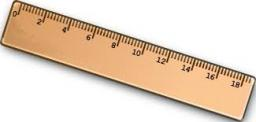# Fraction 4114

Express the fraction and decimal number of which part of the meter is: 120mm, 25cm, 7dm, 670mm, 48cm, 9.7dm

a =  3/25
b =  1/4
c =  7/10
d =  67/100
e =  12/25
f =  97/100

### Step-by-step explanation:Did you find an error or inaccuracy? Feel free to write us. Thank you!

Tips for related online calculators
Need help calculating sum, simplifying, or multiplying fractions? Try our fraction calculator.
Do you want to convert length units?